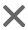# php多图片上传的实现(pbootcms多图上传)AndyGuo2020-06-17 08:06:24PHP692人已围观

```<html>
<body>
<style>
form{
margin: 20px;
}
#picInput>input{
display: block;
margin: 10px;
}
</style>
<form action="pic.php" method="post" enctype="multipart/form-data">
<input type="hidden" name="MAX_FILE_SIZE" value="1000000">
<div id="picInput">
上传图片：<input type="file" name='myfile[]'>
</div>
<input type="submit" value="上传文件">
</form>
<script>
var input = document.createElement("input");
input.type = 'file';
input.name = 'myfile[]';
var picInut = document.getElementById('picInput');
picInut.appendChild(input);
if(picInut.children.length == 3){
}
}
</script>
</body>
</html>```

#### 知识点：

1.通过JS动态添加input结点和设置属性，当大于3个上传图片的时候就不再给予上传图片的机会了。

2.这里最主要的是input输入框的name值，通过动态的添加数组元素，将所有上传的图片动态添加到数组当中。

#### 后端处理功能

Connection.php

1.读取和存储都需要用到连接数据库，所以可以把它封住一下，养成良好的习惯

```<?php
//创建对象并打开连接，最后一个参数是选择的数据库名称
\$mysqli = new mysqli('localhost','root','','test');
//检查连接是否成功
if (mysqli_connect_errno()){
//注意mysqli_connect_error()新特性
die('Unable to connect!'). mysqli_connect_error();
}```

#### pic.php处理功能

```<?php
require_once 'connection.php';
\$file = \$_FILES['myfile'];  //得到传输的数据,以数组的形式
\$name = \$file['name'];      //得到文件名称，以数组的形式
//当前位置
foreach (\$name as \$k=>\$names){
\$type = strtolower(substr(\$names,strrpos(\$names,'.')+1));//得到文件类型，并且都转化成小写
\$allow_type = array('jpg','jpeg','gif','png'); //定义允许上传的类型
//把非法格式的图片去除
if (!in_array(\$type,\$allow_type)){
unset(\$name[\$k]);
}
}
\$str = '';
foreach (\$name as \$k=>\$item){
\$type = strtolower(substr(\$item,strrpos(\$item,'.')+1));//得到文件类型，并且都转化成小写
echo 'success';
}else{
echo 'failed';
}
}
//向指定id插入图片地址（虽然是插入，但是是更新字段，不要迷糊了）
\$uid = 1;
\$str = substr(\$str,1);
\$sql = "UPDATE upload set pic = '".\$str."' WHERE id = ".\$uid;
\$result = \$mysqli->query(\$sql);```

#### 图片显示功能

```<?php
require_once 'connection.php';
\$uid = 1;
\$sql = "SELECT pic FROM upload WHERE id =".\$uid;
\$result = \$mysqli->query(\$sql);
//取出第一个图片的地址
\$picpath = '';
while (\$row = \$result->fetch_array()){
\$picpath = \$row;
}
\$picpath = explode(',',\$picpath);
echo "<img src='".\$picpath."'>";
?>```## 文章评论

• 这篇文章还没有收到评论，赶紧来抢沙发吧~

## 点击排行